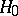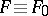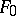# Goodness-of-fit test

(diff) ← Older revision | Latest revision (diff) | Newer revision → (diff)
A statistical test for goodness of fit. The essence of such a test is the following. Letbe independent identically-distributed random variables whose distribution functionis unknown. Then the problem of statistically testing the hypothesisthatfor some given distribution functionis called a problem of testing goodness of fit. For example, ifis a continuous distribution function, then as a goodness-of-fit test for testingone can use the Kolmogorov test.